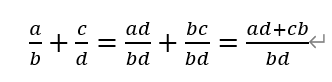# Python面向对象（上）

python是一门面向对象的编程语言。何为对象？对象是类的实例。在生活中，任何一个事物都是一个对象，如牡丹花。牡丹花的类是花类，同样属于花类的还有荷花、月季花、金银花、菊花、梅花、兰花等，它们都是花科，但是每一朵花都有不同的枝叶，不同的色彩，不同的长相。这就是花类的各个属性了，如玫瑰花是红色，菊花是黄色……除了属性，对象里还有方法，也就是花儿怎样开花，怎样长出形态等。

```class Fraction:

def __init__ (self, top, bottom):

self.num = top

self.den = bottom```

`Myfra = Fraction(3, 5)`

#创建了一个对象Myfra，并给予初始值3和5，对应分子分母，所以为三分之五

```def show(self):

print(str(self.num)+“/”+ str(self.den))```

`Myfra.show()`

```def __str__(self):

return str(self.num) +“/ ”+str( self.den))```

```Myfra = Fraction(3, 5)

print(Myfra)```

```def __add__ (self, otherFraction):

newNum = self.num * otherFraction.den + \

self.den * otherFraction.num

newDen = self.den * otherFraction.den

return Fraction(newNum, newDen)``````F1 = Fraction(1,4)

F2 = Fraction(1,2)

F3 = F1 + F2

print(F3)```

```def gcd(m, n):

while m % n != 0:

oldm = m

oldn = n

m = oldn

n = oldm%oldn

return n```

#改良版分数的加法

```def __add__(self, otherFraction):

newNum = self.num * otherFraction.den + \

self.den * otherFraction.num

newDen = self.den * otherFraction.den

common = gcd(newNum, newDen)

return Fraction(newNum // common, newDen // common)```

```def __eq__(self, other):

firstNum = self.num * other.den

secNum = other.num * self.den

return firstNum == secNum```

```class Fraction:
def __init__(self, top, botton):
self.num = top
self.den = botton

def __str__(self):
return str(self.num) + "/" + str(self.den)

newnum = self.num * otherFraction.den + self.den * otherFraction.num
newden = self.den * otherFraction.den

common = gcd(newnum, newden)

return Fraction(newnum // common, newden // common)

def __eq__(self, otherFraction):
firstnum = self.num * otherFraction.den
secnum = self.den * otherFraction.num

return firstnum == secnum

def gcd(m, n):
while m % n != 0:
oldm = m
oldn = n
m = oldn
n = oldm % oldn
return n

if __name__ == '__main__':
f1 = Fraction(3, 4)
f2 = Fraction(3, 4)
f3 = f1 + f2
print(f3)
print(f1 == f2)

f4 = Fraction(4, 8)
f5 = Fraction(2, 4)
print(f4 == f5)
print(f4 + f5)```

posted @ 2023-01-23 21:08  dtes  阅读(142)  评论(0编辑  收藏  举报